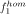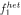A modifying locus with two alleles A1 and A2 B object locus with two alleles B1 and B2two-locus gamete (A i B k ) with allele i at the A- and allele k at the B-locuszygote or any other diploid genotype originating from fusion of ga- metes (A i B k ) and (A j B l ). Note that because of unordered geno- typesbut because of linkagea i , b k relative frequencies of alleles A i , B k among adultsrelative frequency of gametein the gametic productionrelative frequency of genotypeamong adults a ij , b kl relative frequency of genotype A i A j and B k B l , respectively, among adults r recombination frequency (0 ≤ r ≤ ½)probability of fusion when the gametesandencounter. f-values are assumed to differ in only three ways: f2 := probability of fusion in the presence of allele A2 in at least one of the two encountering gametes,for all i, k, l;:= probability of fusion when A1-carriers with equal B-alleles encounter (homotypic mating),;:= fusion probability among A1-carriers with different B- alleles (heterotypic mating),; v kl viabilities at the B-locuscombined selection value of fusion probability and viability selection, defined as